## Friday, 28 September 2007

### Equations of Extrusion

When I first tested my extruder I found that the filament diameter varied with the flow rate and temperature. This was contrary to what others have experienced so I decided to investigate further. It turns out that this is known as die swell and is caused by non Newtonian fluids expanding after they have been squeezed through a hole. Apparently it is a very complicated subject.

To get an idea of what was going on I designed my extruder controller to be able to make measurements. Rather than drive the motor with open loop PWM I used a shaft encoder with proportional feedback. Instead of specifying what PWM setting to use, the host specifies how many shaft encoder steps to move and at what rate. The extruder controller then adjusts the PWM to maintain the correct shaft position at any given instant. Assuming the filament does not slip against the drive screw, that means I can extrude a known volume of plastic in a known time to the tolerance of the the original feed material. The host can then ask the controller what the total on time and off times have been so that it can calculate the average power that has been used.

My temperature control works in a similar way. The host calculates the resistance the thermistor should have at the desired temperature, and from that, what voltage reading the ADC should produce. It sends that setting to the controller which turns the heater on and off. Again it keeps track of the total on and off times so that the host can calculate the average power.

My heater has a resistance of 8.5Ω and has 11.8V across it after the drop in the MOSFET switch and the wiring. That gives a power of 16.4W. This is a graph of the temperature reading from the thermistor plotted against the heater duty cycle :-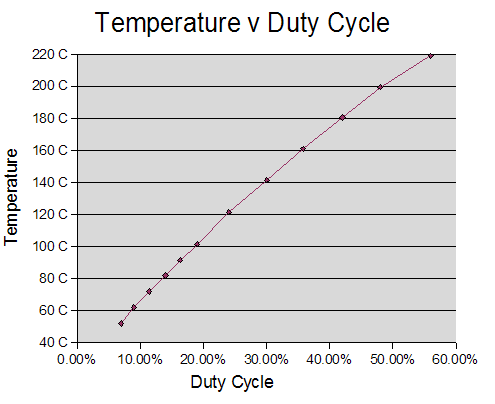As you can see it is not quite a straight line. This is because the resistance of the nichrome heating element increases slightly as it gets hotter, so power does not quite rise in line with the duty cycle. I measured the resistance at 200°C to be 9.7Ω. Using the formula:

R = R0[1 + α(TT0)]

that gives a temperature coefficient α of 7.8 × 10-4 which is about twice the figure I found on the web for nichrome. I expect that it varies widely according to the exact alloy being used.

Here is a graph of temperature against power, calculated using the above formula for resistance :-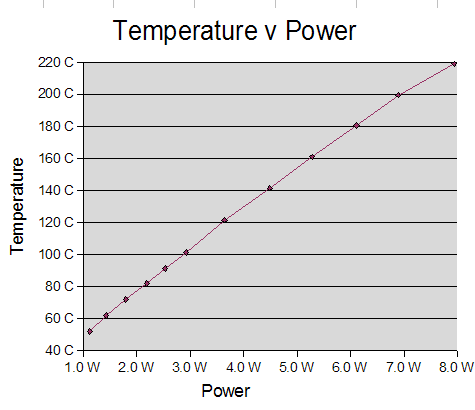It is a lot closer to the straight line I was expecting.

I decided to investigate how much extra power is needed to heat the incoming plastic when extruding. I found that while feeding the filament in at 1mm/s, which is about the maximum my motor can do, the PWM to maintain 200°C increased from 44.6% to 61.2%. An increase of 16.6% corresponding to an extra 2.4W. Feeding a 3mm filament at 1mm/s gives a flow rate of 7.1 × 10-3 cc/s. HDPE has a density of around 1 so that is 7 × 10-3 g/s. The specific heat capacity is 2.2 J/g-°C which gives 2.8W to heat 7 mg from 20°C to 200°C per second. I think that is reasonably close to the value I measured, given that HDPE has quite a wide range of densities.

Next I decided to look at the effect of temperature on the motor power required to extrude at a given rate :-I concluded that temperature has little effect on the motor power required, except when it gets close to the melting point, where it rises rapidly as expected. That was how I broke my extruder!

Next I looked at filament diameter against temperature :-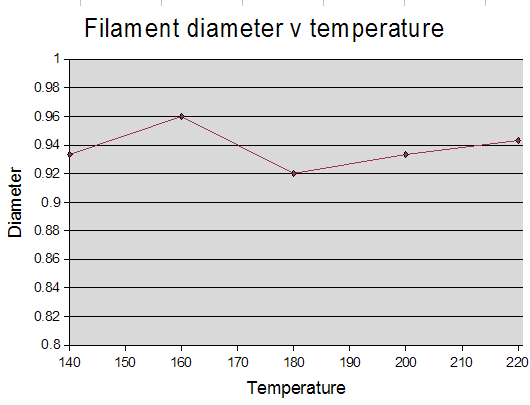No real correlation, so it seems temperature is not very important as long as it is above the melting point. This was a surprise to me as I imagined molten plastic would get less viscose as temperature increased. It may become more critical when I start laying down filament as it will effect how it fuses together and shrinks. I did all the subsequent measurements at 200°C.

Feed rate (in mm/s) against PWM was another surprise. I expected power to rise rapidly with feed rate but, in fact, it is quite proportional :-Presumable 30% is the power required to overcome static friction in the system.

Here you can see the output rate versus the feed rate :-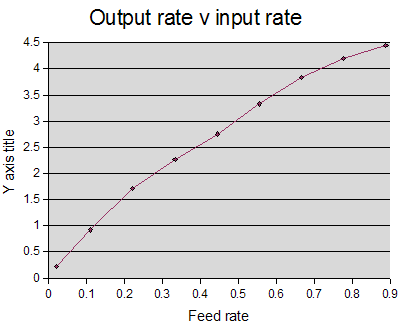It does not increase in proportion, so if conservation of matter is true then it must be getting bigger in diameter. Indeed it does, here is output diameter against output rate :-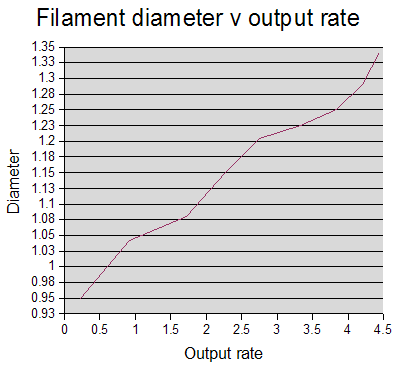Either it is a very complex relationship with multiple inflexions or it is just linear with lots of measurement error. I made three measurements per test with digital calipers and took the average but the deviation between samples was quite high.

I prefer to think it is a simple linear relationship which means I can make a simple mathematical model of my extruder. As you can see it will hit the Y axis at about 0.93 mm. I think that must be the size of the hole in my nozzle. I drilled it 0.5mm but perhaps I drilled the hole from the back too far and opened it out a bit. It seems to have got bigger with use because I could get 0.8mm filament when I first tested it but I don't seem to be able to now, even at very low extrusion rates.

So if the filament diameter equals hole size plus a constant times extrusion rate then from conservation of volume I can relate the output rate to the feed rate.

do = dh + kvo

vodo2 = vidi2

So: vo(dh + kvo)2 = vidi2 a cubic equation!

Where do is the output filament diameter, di is the input filament diameter, dh is the nozzle hole diameter and vo is the output filament speed, vi is the input filament speed.

With these equations I can calculate the output rate to get a particular filament diameter. That also tells me how fast to move the head. From the output rate I can also calculate the feed rate required.

Conclusion? Well I definitely have die swell which increases with extrusion rate but other people have reported constant die swell. The only explanation I can think of is that I drilled my nozzle too deep from the back so the aperture has almost zero thickness instead of the 0.5 to 1mm expected.

I have a simple mathematical model which allows me to exploit the variable filament width if I need to. This may all become irrelevant when I start laying down filament to build things because the filament can be stretched or compressed if the head movement does not match the output rate.

Tomorrow I will try laying down the filament.

1.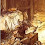2.